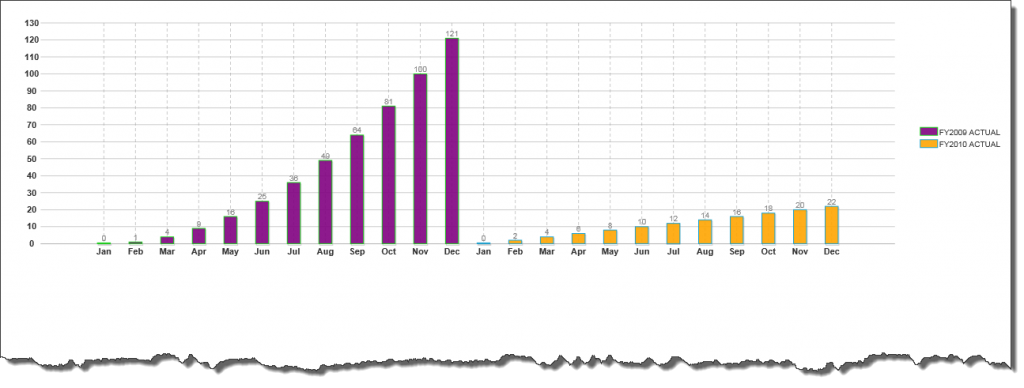﻿ Customized ValueLabels in Wijmo C1BarChart# Customized ValueLabels in Wijmo C1BarChart

C1BarChart is one of the most extensively used tool available in the Wijmo controls. In addition to its feature rich interface, it also provides flexibility to developers to work with client side code to get the desired results. Typically when a series is added to C1BarChart, the X and Y axis automatically picks up the labels and display them. However there might be situations when we wish to display some custom text as the chart labels rather than the default text. In this blog article we will see how ValueLabels class comes into play when custom labels are needed for the chart. We will end up creating a chart which will look like this:A two series Bar chart with custom X Axis labels

So basically we are creating a two series bar chart which shows data according to months. The first thing we need to do is to set up data for both the series. The following code provides an example of doing it.

``````#region Series Addition
double[] unitsSold = new double;
double[] month = new double;

//populate the array
int i = 0;
for (i = 0; i < 12; i++)
{
unitsSold[i] = i;
month[i] = i * i;
}

//create an object of chart series and add data
BarChartSeries bcs = new BarChartSeries();
bcs.Label = "FY2009 ACTUAL";

double[] unitsSold1 = new double;
double[] month1 = new double;
//populate the array
int j = 0;
int counter = 0;
for (j = 12; j < 24; j++)
{
unitsSold1[counter] = j;
month1[counter] = counter * 2;
counter++;
}

//create an object of chart series and add data
BarChartSeries bcs1 = new BarChartSeries();
bcs1.Label = "FY2010 ACTUAL";

#endregion
``````

So this takes care of the data which will be used to plot the chart. The next thing we need to do is to set the custom labels for the X-Axis. Since we are going to display month names, we will store all the month names in an array and then loop through them to add them to the X-Axis value label list. Here is the code we require:

``````#region Adding Value Labels
//array consisting names of the months
string[] monthNames = DateTimeFormatInfo.CurrentInfo.MonthNames;
//FOR THE FIRST SERIES
for (i = 0; i < monthNames.Length - 1; i++)
{
//create object of ValueLabel class
ValueLabel vl = new ValueLabel();
// set the x-axis value which you want to replace
vl.NumericValue = i;
// set the desired value which you want to show
vl.Text = monthNames[i].Substring(0, 3);
// add it to the ValueLabel collection
}

//set the annotation to valuelabels instead of values.
C1BarChart1.Axis.X.AnnoMethod = ChartAxisAnnoMethod.ValueLabels;

//FOR THE SECOND SERIES
for (i = 0; i < monthNames.Length - 1; i++)
{
//create object of ValueLabel class
ValueLabel vl = new ValueLabel();
vl.NumericValue = i + 12;
// set the desired value which you want to show
vl.Text = monthNames[i].Substring(0, 3);

// add it to the ValueLabel collection
}

//set the annotation to valuelabels instead of values.
C1BarChart1.Axis.X.AnnoMethod = ChartAxisAnnoMethod.ValueLabels;
#endregion
``````

In addition to this, we can add few lines of code to make some cosmetic changes to the chart; however I have omitted that part since we are focusing on customizing the value labels. So we have all the code needed to customize the value label as per our requirements. A sample application which demonstrates this implementation can be downloaded from the link below. Download C# SampleGrapeCity

GrapeCity Developer Tools